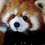# 1/2 + 1/3 + 1/5 + 1/7 + 1/11 + 1/13 ...

We're going to look at how the infinite sum of prime reciprocals behaves. To start off, let's take a closer look at the harmonic series, or the sum of the reciprocals of all numbers: $\displaystyle\sum_{n=1}^\infty \frac{1}{n} = \frac{1}{1} + \frac{1}{2} + \frac{1}{3} + \frac{1}{4} + \cdots = \infty$

We can show that this sum diverges by comparing this to the following Taylor series

$ln\left(\frac{1}{1-x}\right) = \displaystyle\sum_{n=1}^\infty \frac{x^{n}}{n}$

Evaluating the limit at $x=1$

$ln(\infty) = \displaystyle\sum_{n=1}^\infty \frac{1}{n} = \infty$

If this feels hand-wavy, that's because it is. However this result still turns out to be valid when more careful work is done. But how can this sum tell us anything about the sum of prime reciprocals? To start off, the fundamental theorem of arithmetic tells us that any integer $n$ can be written uniquely as a product of prime numbers, or $n = p_{1}^{k_{1}}p_{2}^{k_{2}}p_{3}^{k_{3}}...$, where $k_{n}$ is either $0$ or a positive integer. This uniqueness leads to the next equation

$\displaystyle\sum_{n=1}^\infty \frac{1}{n} = \displaystyle\prod_{p=p_{1}}^\infty (1 + \frac{1}{p} + \frac{1}{p^{2}} + \frac{1}{p^{3}} + \cdots)$

Essentially, this is a product over all powers of all prime numbers. Thanks to the fundamental theorem of arithmetic, we know that all possible combinations of primes and their powers will lead to all possible whole numbers. Thus, this formula is valid. Using a Taylor series allows us to simplify this expression to

$\displaystyle\sum_{n=0}^\infty x^{n} = \frac{1}{1-x} \quad x<1$ $\displaystyle\prod_{p=p_{1}}^\infty (1 + \frac{1}{p} + \left(\frac{1}{p}\right)^{2} + \left(\frac{1}{p}\right)^{3} + \cdots) = \displaystyle\prod_{p=p_{1}}^\infty \frac{1}{1-p^{-1}}$

Taking the logarithm of the last expression yields

$ln\left(\displaystyle\sum_{n=1}^\infty \frac{1}{n}\right) = ln(ln(\infty)) = \displaystyle\sum_{p=p_{1}}^\infty ln\left(\frac{1}{1-p^{-1}}\right)$

Using the Taylor series we used in the beginning of this note, we can turn the last equality into

$ln(ln(\infty)) = \displaystyle\sum_{p=p_{1}}^\infty \left(\frac{1}{p} + \frac{1}{2p^{2}} + \frac{1}{3p^{3}} + \cdots \right) \sim \displaystyle\sum_{p=p_{1}}^\infty \frac{1}{p}$

We can ignore the higher-order terms because they are bounded by $\zeta(n)$, which converges to a finite value for $n>1$. Finally, we get

$\displaystyle\sum_{p=p_{1}}^\infty \frac{1}{p} =ln(ln(\infty)) = \infty$Note by Levi Walker
2 years, 8 months ago

This discussion board is a place to discuss our Daily Challenges and the math and science related to those challenges. Explanations are more than just a solution — they should explain the steps and thinking strategies that you used to obtain the solution. Comments should further the discussion of math and science.

When posting on Brilliant:

• Use the emojis to react to an explanation, whether you're congratulating a job well done , or just really confused .
• Ask specific questions about the challenge or the steps in somebody's explanation. Well-posed questions can add a lot to the discussion, but posting "I don't understand!" doesn't help anyone.
• Try to contribute something new to the discussion, whether it is an extension, generalization or other idea related to the challenge.
• Stay on topic — we're all here to learn more about math and science, not to hear about your favorite get-rich-quick scheme or current world events.

MarkdownAppears as
*italics* or _italics_ italics
**bold** or __bold__ bold
- bulleted- list
• bulleted
• list
1. numbered2. list
1. numbered
2. list
Note: you must add a full line of space before and after lists for them to show up correctly
paragraph 1paragraph 2

paragraph 1

paragraph 2

[example link](https://brilliant.org)example link
> This is a quote
This is a quote
    # I indented these lines
# 4 spaces, and now they show
# up as a code block.

print "hello world"
# I indented these lines
# 4 spaces, and now they show
# up as a code block.

print "hello world"
MathAppears as
Remember to wrap math in $$ ... $$ or $ ... $ to ensure proper formatting.
2 \times 3 $2 \times 3$
2^{34} $2^{34}$
a_{i-1} $a_{i-1}$
\frac{2}{3} $\frac{2}{3}$
\sqrt{2} $\sqrt{2}$
\sum_{i=1}^3 $\sum_{i=1}^3$
\sin \theta $\sin \theta$
\boxed{123} $\boxed{123}$

## Comments

There are no comments in this discussion.

×

Problem Loading...

Note Loading...

Set Loading...